Question

In the calibration solutions, the very large excess of Fe3+ ion compared to the amount of NCS- ion used to prepare the the mixtures allows us to make what assumption about the concentration of product in the mixtures? Question 8 options:

[Fe(NCS)2+]equilibrium = [NCS-]equilibrium

[Fe(NCS)2+]equilibrium = [Fe3+]initial

[Fe(NCS)2+]initial = [Fe3+]initial

[Fe(NCS)2+]equilibrium = [Fe3+]equilibrium

 [Fe(NCS)2+]equilibrium = [NCS-]initial [Fe(NCS)2+]initial = [NCS-]initial

Ans: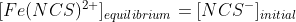The standard solution contains a very large concentration of Fe3+ ([Fe3+]i) and a small initial concentration of SCN ([SCN]i). (The [Fe3+] in the standard is 100 times more than [Fe3+] in the trials.) The high [Fe3+] forces the reaction far to the right, using up nearly 100% of the SCN ions. Therefore, for every one mole of SCN reacted, one mole of FeNCS2+ is produced and [FeNCS2+]std can be assumed equal to [SCN]i, the initial concentration of SCN in the standard solution.

#### Earn Coins

Coins can be redeemed for fabulous gifts.

Similar Homework Help Questions
• ### Lab Report: Determination of Kc for a Complex Ion Formation tube 2.00e-3 Fe3+ (mL) 2.00E-3M SCN-...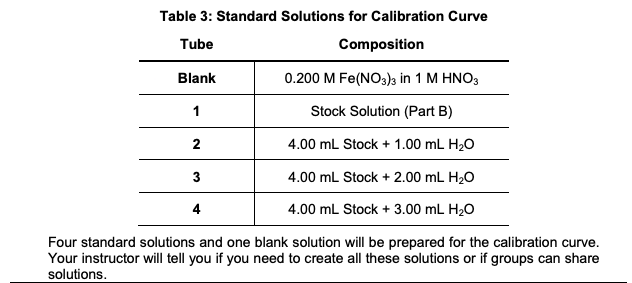Lab Report: Determination of Kc for a Complex Ion Formation tube 2.00e-3 Fe3+ (mL) 2.00E-3M SCN- (mL) water (mL) initial conc. Fe3+ initial conc. SCN- 1 5.00 5.00 0 1.00e-3M 1.00E-3M 2 5.00 4.00 1.00 1.00E-3M 8.00E-3M 3 5.00 3.00 2.00 1.00E-3, 6.00E-3M 4 5.00 2.00 3.00 1.00E-3M 4.00E-3M 5 5.00 1.00 4.00 1.00E-3M 2.00E-3M 10ml of 0.200M Fe3+, 2.00ml of 0.00200M SCN-, AND 8.00ml of water results in an eq. [FeSCN2+] IN Standard Soln.:2.00E-4M Could you please explain how...

• ### Table A. Preparation of Standard solutions of FeSCN2+ 1.0 M HNO3 0.002 M 0.200 M Solution...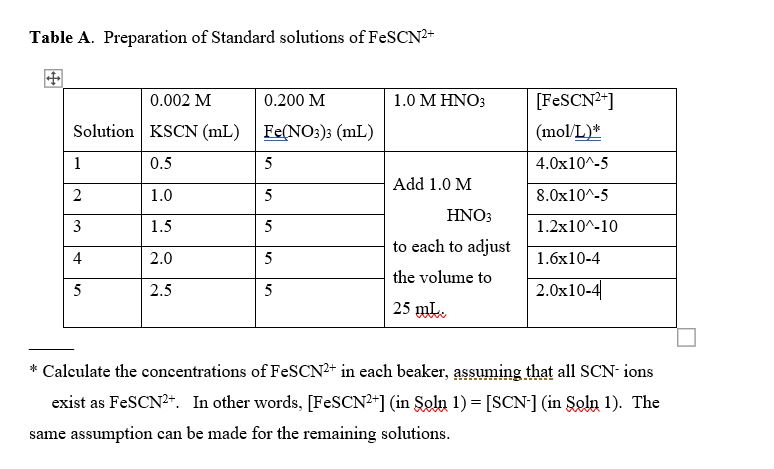Table A. Preparation of Standard solutions of FeSCN2+ 1.0 M HNO3 0.002 M 0.200 M Solution KSCN (mL) Fe(NO3)3 (mL) 0.5 5 [FeSCN2+] (mol/L)* 1 4.0x10^-5 Add 1.0 M 2 1.0 5 8.0x10^-5 HNO3 3 1.5 5 1.2x10^-10 4 2.0 5 1.6x10-4 to each to adjust the volume to 25 mL. 5 2.5 5 2.0x10-4 * Calculate the concentrations of FeSCN2+ in each beaker, assuming that all SCN-ions exist as FeSCN2+. In other words, [FeSCN2+] (in Soln 1) = [SCN-]...

• ### 3. Experimental Procedure, Part A.1. For preparing a set of standard solutions of FeNCS, the equilibrium...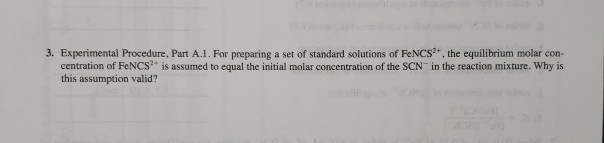3. Experimental Procedure, Part A.1. For preparing a set of standard solutions of FeNCS, the equilibrium molar con- centration of FeNCS is assumed to equal the initial molar concentration of the SCN in the reaction mixture. Why is this assumption valid? A. A Set of Standard Solutions to Establish a The set of standard solutions is used to determine the absorbance of known molar con- centrations of FeNCS2. A plot of the data, known as a calibration curve, is used...

• ### I had clearer images. Part 1 - Making Standard solutions. 1. Into a clean, dry beaker...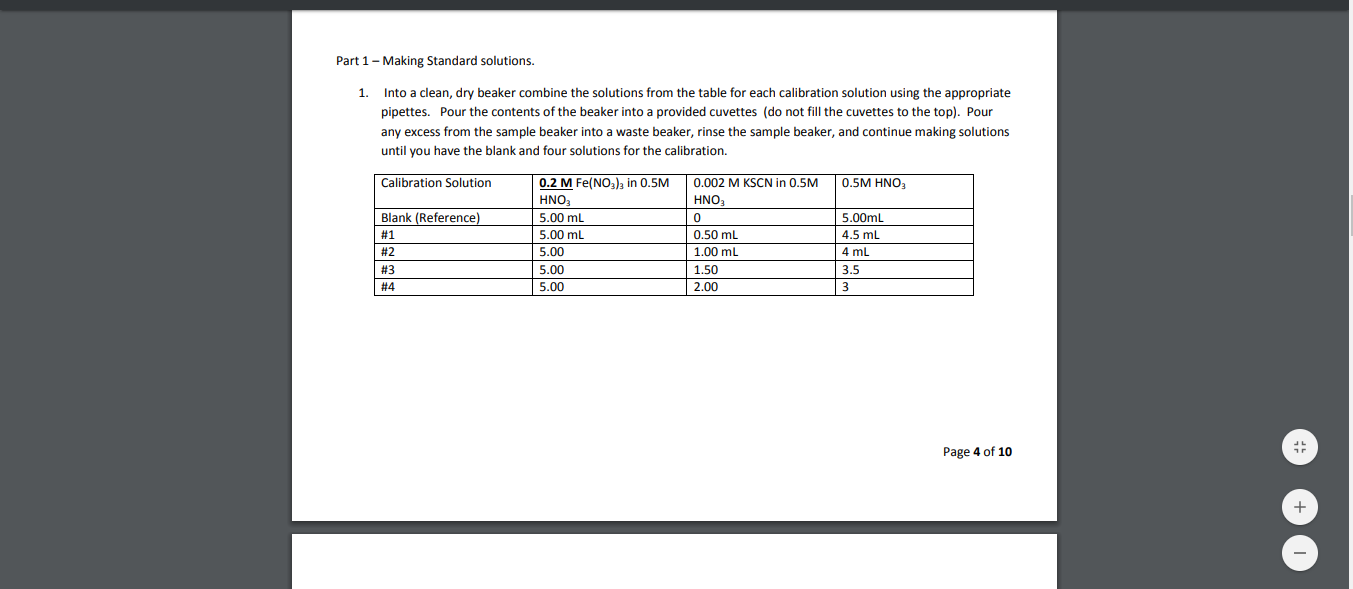I had clearer images. Part 1 - Making Standard solutions. 1. Into a clean, dry beaker combine the solutions from the table for each calibration solution using the appropriate pipettes. Pour the contents of the beaker into a provided cuvettes (do not fill the cuvettes to the top). Pour any excess from the sample beaker into a waste beaker, rinse the sample beaker, and continue making solutions until you have the blank and four solutions for the calibration. Calibration Solution...

• ### Doing a Chem Review, these are just study scenarios that I need to have a good...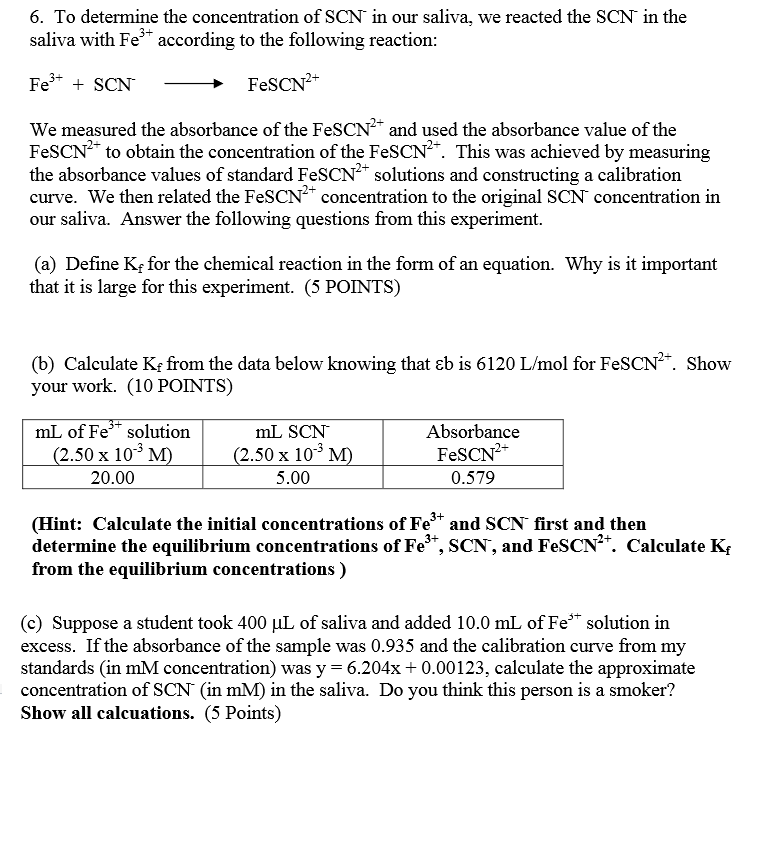Doing a Chem Review, these are just study scenarios that I need to have a good understanding of 6. To determine the concentration of SCN in our saliva, we reacted the SCN in the saliva with Fe' according to the following reaction: We measured the absorbance of the FeSCN and used the absorbance value of the FesCN2 to obtain the concentration of the FesCN2*. This was achieved by measuring the absorbance values of standard FeSCN2+ solutions and constructing a calibration...

• ### please correct me If I did anything wrong. im very confused on this. • Determining k...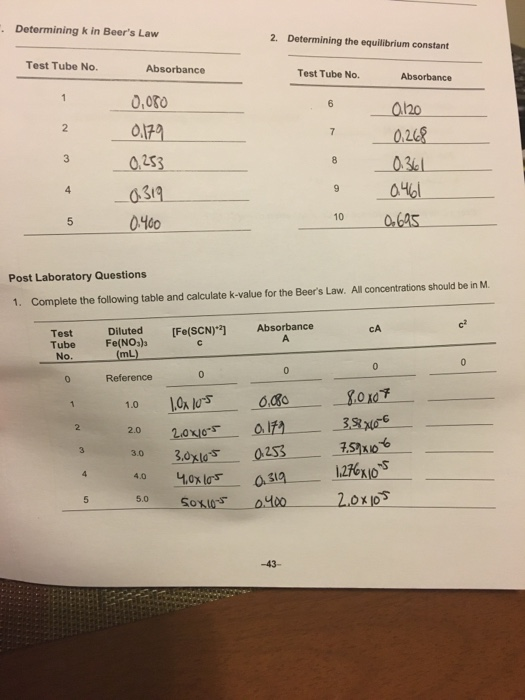please correct me If I did anything wrong. im very confused on this. • Determining k in Beer's Law 2. Determining the equilibrium constant Test Tube No. Absorbance Test Tube No. Absorbance 0,060 이79 0.253 0319 0.400 0.120 0.268 0.361 0.461 0.695 Post Laboratory Questions 1. Complete the following table and calculate k-value for the Beer's Law. All concentrations should be in M. [Fe(SCN)2) Absorbance CA Test Tube No. Diluted Fe(NO3)2 (mL) 0 . 2 3 4 s Reference 1.0...

• ### values used for table 2 how do you do the Ice tables??? Experiment V. Equilibrium Constant...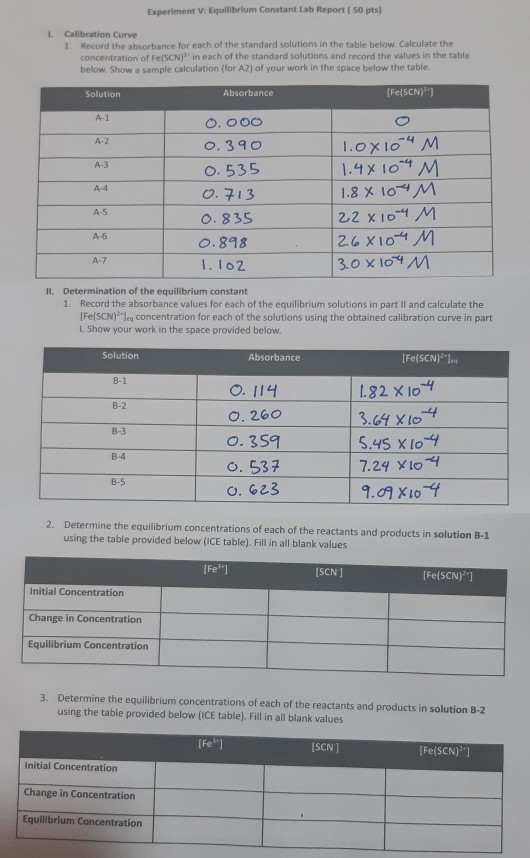values used for table 2 how do you do the Ice tables??? Experiment V. Equilibrium Constant Lab Report ( 50 pts) Calibration Curve 1. Record the absorbance for each of the standard solutions in the table below. Calculate the concentration of FeSCN)" in each of the standard solutions and record the values in the table below. Show a sample calculation (for A2) of your work in the space below the table. Solution Absorbance [Fe(SCN)" A-1 A-2 A-3 0.000 0.390 0.535...

• ### Calculate the initial concentration of Fe3+ in test tubes 1-4. (you will need to take the...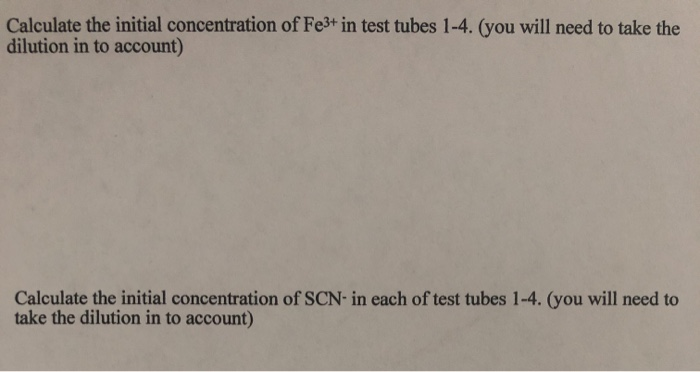Calculate the initial concentration of Fe3+ in test tubes 1-4. (you will need to take the dilution in to account) Calculate the initial concentration of SCN-in each of test tubes 1-4. (you will need to take the dilution in to account) 2. Label four 20 x 150 mm test tubes 1-4. Pour about 30 mL of 0.0020 M Fe(NO3)3 into a clean, dry 100-ml beaker. Pipet 5.0 mL of this solution into each of the four labeled test tubes. Use...

• ### Beers law solve for E3 and E4 showing work Part II. Equilibrium Constant Calculations Calculate the...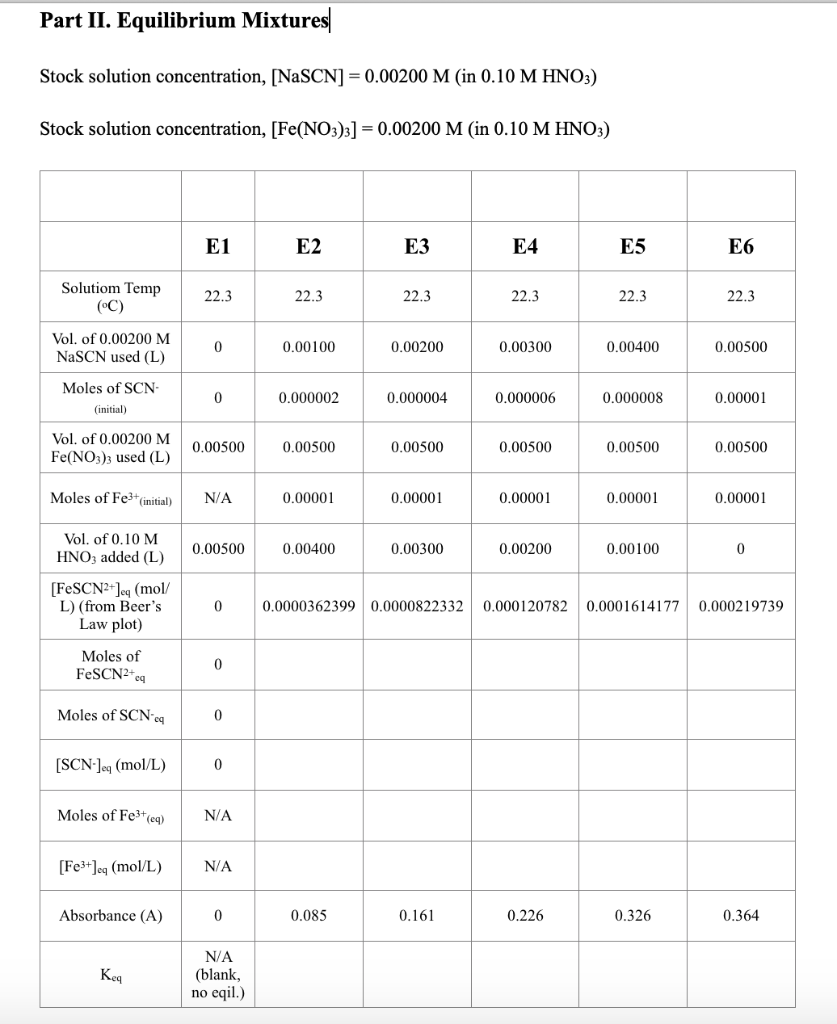Beers law solve for E3 and E4 showing work Part II. Equilibrium Constant Calculations Calculate the initial moles of Fe3+ and SCN- for solutions E2 through E6 and record these values in the table on your Data Sheet. You obtain the moles of the reactants by multiplying their molarity by the volume (in L) of their solution used. Determine the equilibrium concentration of FeSCN2+ for each of the solutions E2-E6 from your Beer’s Law plot. Using these equilibrium concentrations, the...